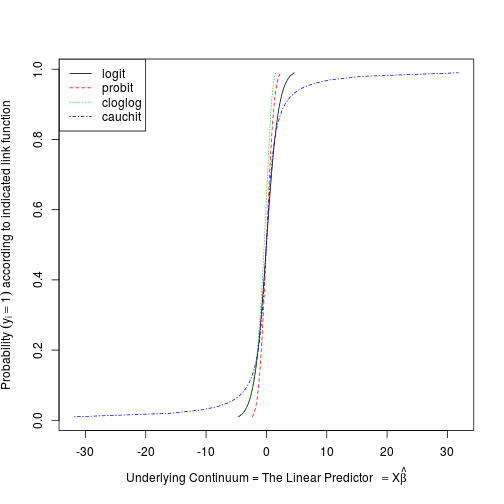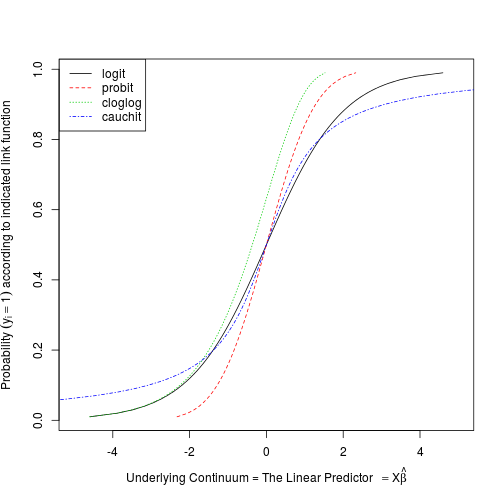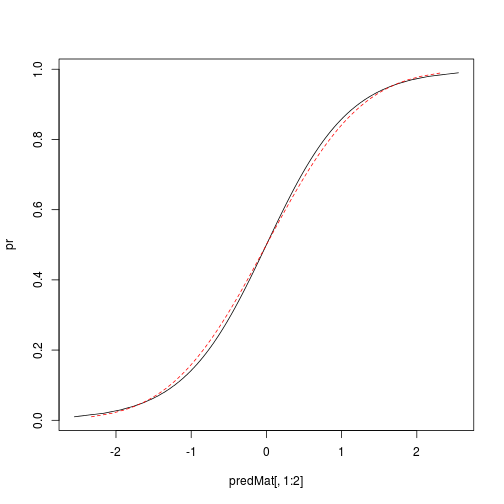``````## Title: logit-compareLinks-1.R
## Author:  Paul E Johnson <pauljohn@ku.edu>
## Date:  2013-02-11 / 2014-08-07
## Description: I started to wonder what difference it might
##   make if we change the link function in a logistic regression.
##   Theoretically, we know that changing from logit to probit
##   has no meaningful difference--the coefficients scale by 1.6
##
## The main take-away for me is that the Cauchit link is WAY
## different from the others.

## For parameters lying between 0 and 1 (e.g., probabilities), the
##   ‘logit’, ‘probit’, ‘cloglog’, ‘cauchit’, ‘loglog’, ‘fsqrt’,
##   ‘logc’, ‘golf’, ‘polf’, ‘nbolf’.

library(VGAM) ## for the logit function
``````
``````## Loading required package: methods
``````
``````pr <- seq(0.01, 0.99, by = 0.01)

## see the logit function?
logit(pr)
``````
``````##   -4.59512 -3.89182 -3.47610 -3.17805 -2.94444 -2.75154 -2.58669
##   -2.44235 -2.31363 -2.19722 -2.09074 -1.99243 -1.90096 -1.81529
##  -1.73460 -1.65823 -1.58563 -1.51635 -1.45001 -1.38629 -1.32493
##  -1.26567 -1.20831 -1.15268 -1.09861 -1.04597 -0.99462 -0.94446
##  -0.89538 -0.84730 -0.80012 -0.75377 -0.70819 -0.66329 -0.61904
##  -0.57536 -0.53222 -0.48955 -0.44731 -0.40547 -0.36397 -0.32277
##  -0.28185 -0.24116 -0.20067 -0.16034 -0.12014 -0.08004 -0.04001
##   0.00000  0.04001  0.08004  0.12014  0.16034  0.20067  0.24116
##   0.28185  0.32277  0.36397  0.40547  0.44731  0.48955  0.53222
##   0.57536  0.61904  0.66329  0.70819  0.75377  0.80012  0.84730
##   0.89538  0.94446  0.99462  1.04597  1.09861  1.15268  1.20831
##   1.26567  1.32493  1.38629  1.45001  1.51635  1.58563  1.65823
##   1.73460  1.81529  1.90096  1.99243  2.09074  2.19722  2.31363
##   2.44235  2.58669  2.75154  2.94444  3.17805  3.47610  3.89182
##   4.59512
``````
``````linknames <- c("logit", "probit", "cloglog", "cauchit")
##could try , "fsqrt", "logc", "golf")

predMat <- sapply ( linknames, do.call, list(pr))

## Lets just throw all the plots into one file.

SAVEME <- FALSE
if (SAVEME == TRUE) ## Same as if (SAVEME)
pdf(file = "logitlinks.pdf", height = 7, width = 7,
paper = "special", onefile = TRUE,family="Times")

myxlab <-
expression(paste("Underlying Continuum = The Linear Predictor",
phantom(0) == X*hat(beta)))

myylab <- expression(paste("Probability ", (y[i]==1),
" according to indicated link function"))

## Here's the easy way to make the  plot
matplot(predMat, pr,  type="l", xlab = myxlab, ylab=myylab)
````````````## re-scale a bit
matplot(predMat, pr,  type = "l", xlab = myxlab, ylab = myylab, xlim = c(-5, 5))
legend("topleft", legend = linknames, col = 1:4, lty = 1:4)
````````````## Here's the way I did it when I had forgotten about matplot.
## Maybe there is some virtue in hard work. I think the result
## is nicer because the scaling is better. The scaling is set
## by the first function plotted, so the huge domain of the
## cauchit distribution is not distorting the display of the others.

## This plots column 1, then adds lines for columns 2:4

plot(predMat[, 1], pr, type = "l", cex = 0.5, xlab = myxlab, ylab =
myylab)
## That sets the outer frame. Now for columns 2 through 4
## Could be more careful using "raw" indexes and position numbers
for(i in 2:4){
lines(predMat[, i], pr, type ="l", lty = i)
points(predMat[90, i], pr, cex = 2)
points(predMat[90, i], pr, pch = as.character(i))
points(predMat[10, i], pr, cex = 2)
points(predMat[10, i], pr, pch = as.character(i))
}

````````````if (SAVEME == T) dev.off()

## But, as I look at this, I can see a big flaw in the plot.  Since
## any fit of a logit or probit model will stretch or implicitly
## rescale the X axis, the difference that appears here between probit
## and logit is a deception. It comes back to the fact that probit and
## logit coefficients are proportional, their seeming difference is
## an illusion (translate  by multiplying by 1.7 or such)

## TODO: Adjust to plot predicted values using a rescaled
## X axis.  Hmm. I have to think that over.

## Logit has a scale parameter s, variance is  pi^2/3 * s^2
## The fitting process forces the error to be 1, however,
## so the betas are actually magnified.
## Probit has variance 1

## Let's rescale column 1 appropriately

predMat[,1] <- predMat[ ,1]/ 1.8

## now just look at logit and probit. See? same. almost.
matplot( predMat[, 1:2], pr,  type = "l")
````````````## How now to rescale Cauchy?
``````